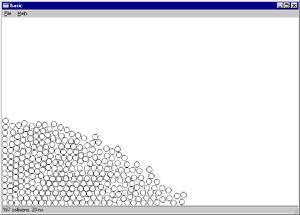# Fluid Simulation

Written by Peter Birtles
October 2000There are N balls, all of the same size and mass. Only one ball can occupy the same area in space. The balls are contained by 4 limits - xmax, xmin, ymax, ymain - which correspond to the edges of a GUI window. Events such as a window resize or move alter these 4 limits. In effect the boundary conditions change. I also use the window's REPAINT message as the main display loop trigger.The basic outline of the processing loop is as follows:

```friction = 0.95
timeconstant = 1.0 ; = 2.0 for faster, less accurate behaviour, etc.
a ball's mass = 1.0 unit

For each display iteration -

/* work out physics, not exactly but rough enough to make it "look" fun */
1/ detect all collisions , a N squared job with my naive code
for all balls A
for all balls B
if A&B are close enough to collide then
- work out the force that ball A will apply to ball B
and add this force divided by B's mass to B's acceleration
- work out the force that ball B will apply to ball A
and add this force divided by A's mass to A's acceleration
Some consideration is given to how closely they collide and
the closer the collision, the more force is applied.

2/ integrate acceleration
vx = vx * friction + ax * timeconstant
vy = vy * friction + ay * timeconstant

3/ then integrate velocity
px = px + vx * timeconstant
py = py + vy * timeconstant

4/ apply boundry conditions (in a very real sense too...)

in the case of the xmin limit -
for all balls
if px < xmin then
px = xmin
vx = -vx	i.e. bounce back in other direction
end if
and similarly for the other three boundaries.

/* update display */
for all balls,
erase ball at previous position (opx,opy)
draw ball at current position (px,py)
set old position = current position (i.e. opx = px, opy = py)

next display iteration
```

When the window is selected by the title bar, the user can drag the window (at surprising speeds) all over the GUI screen area. The balls behave like they have inertia, backing up against the the trailing window edge as the window moves. When the window stops, the balls then show they are primarily under the influence of gravity. And so they settle down, stacking themselves quite neatly Resizing the window also has similar effects.

I have added a bit of human interest. The user can select a ball with the mouse. Once selected it is now drawn in red and follows the motion of the mouse pointer. The user can bash the red ball into a stack of motionless balls and see what happens. (balls flying everywhere.) Or subtle changes can be made to the stacking, inserting dislocations etc into the ordered stack. I think this could be a bit of help in elementary crystallography lessons.

The screen shots I sent you have 300 balls, each 14 pixels in diameter. The update speed on my Pentium 3 450Mhz, running Win98 is about 20 milliseconds. This is barely enough for a gratifying interactive experience. Most of the time is spent either drawing circles or in the collision detection loop (with an N squared computing cost too).

"Fluid" type behaviour is seen when a large stack of balls which was previously closely contained is allowed to spread out over a larger flat area. The first few balls to arrive at the limits of the new area seem to have a lot more speed and tend to splash about a bit. An easy way to make this happen is to resize the window fairly small, let things settle down and then drag a boundary quickly to the left or right

 Windows Binaries MSWindows binaries are given here: balls.zip for a 200 and 400 ball system. Source code The windows C code can be found here. All of the GUI and OS specific code is fairly easy to spot.Three important procedures to look out for are

• create_balls() which initializes the balls with some sort of position in space, zero speed and zero acceleration.

• display_balls() which for each ball erases the old position and draws it in it's new position.

• update_balls() which does the physics as explained above.

The variable "got_one" = 0 when no ball is under the mouse control and it equals the ball's index when a ball has been selected by the mouse and is under mouse control.